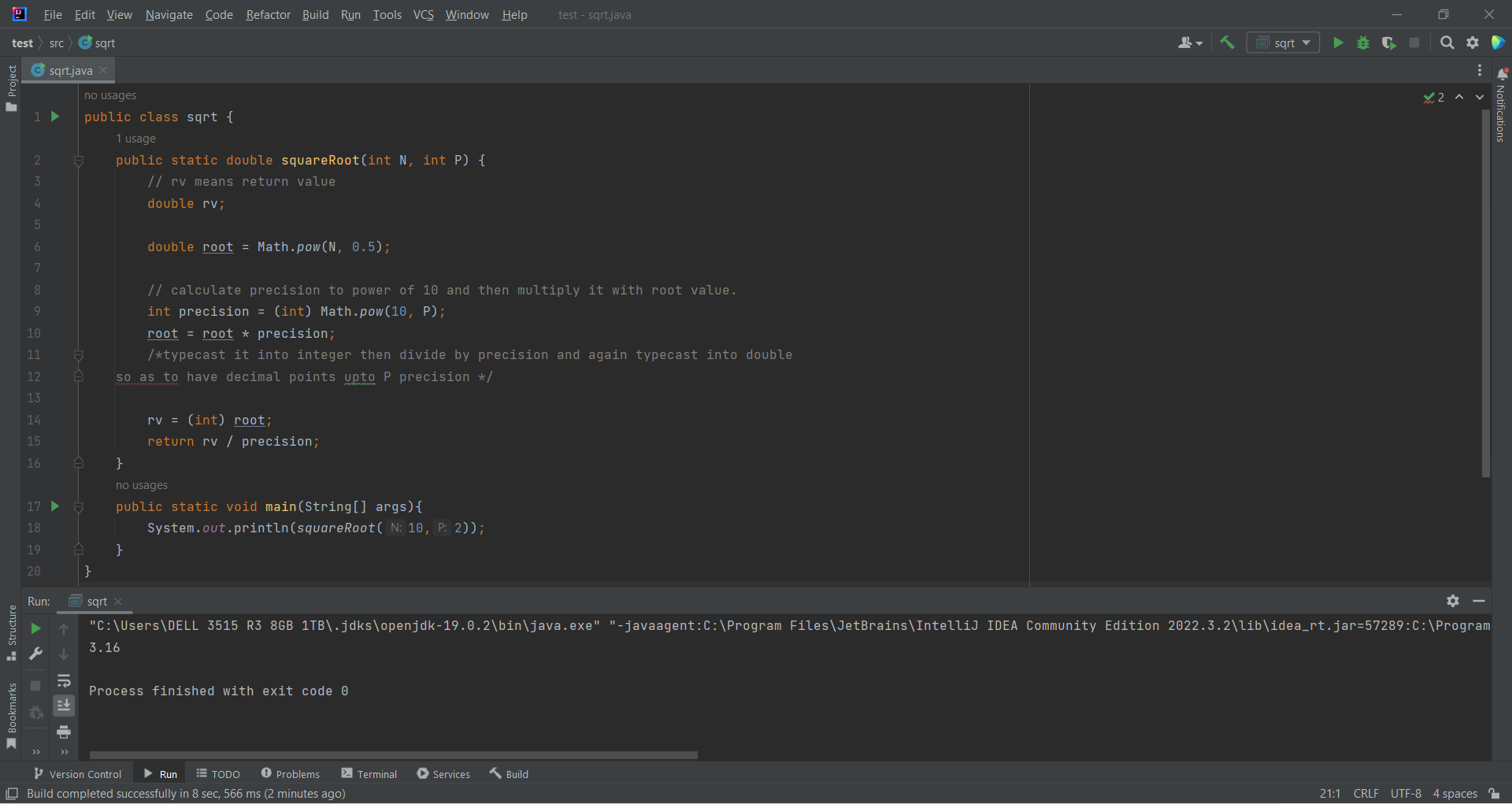# Calculate the Square Root of an Integer Number in Javaby Abdul Rawoof A RUpdated: Mar 7, 2023Guide Kit

In Java, a square root of a number is a value that gives the number when multiplied by itself. For example, 9 x 9 = 81, so a square root of 81 is 9. Note that (-9) x (-9) = 81, too, so -9 is also a square root of 81.

The formula for the square root is an inverse method of squaring a number. Hence, squares and squares roots are corresponding concepts. Suppose x is the square root of y. Then it represents x=√y. In another way, we can express the same equation as x²=y. The radical symbol used to represent the root of the numbers is '√. ' Java has one important method called sqrt(), which returns the square root of a type value double passed to it as an argument. If the argument is NaN or negative, the result is NaN. The result is positive infinity when the argument is positive infinity. The result will be the same as the argument if the argument passed is positive zero or negative zero.

We have two different ways to square a number in Java:

1. Call the Math. pow function.
2. Multiply the number by itself.

Here is an example of how to calculate the square root of an integer number in Java:Fig : Preview of the output that you will get on running this code from your IDE.

### Instructions

Follow the steps carefully to get the output easily.

1. Install IntelliJ IDEA IDE on your computer.
2. Create a new project then create a class file.
3. Copy the code snippet using 'copy' button and paste it in that class file.
4. Create a main method at end of the code after pasting, then call the class method in main method(refer preview of the output for your reference).
5. Run the file to generate the output.

I hope you found this useful.

I found this code snippet by searching for 'Calculate the square root of an integer number' in kandi. You can try any such use case!

### Environment Tested

I tested this solution in the following versions. Be mindful of changes when working with other versions.

1. The solution is created in IntelliJ IDEA 2022.3.2.
2. The solution is tested on Java 19(Oracle JDK 19).

Using this solution, we are able to calculate the square root of an integer number in java with simple steps. This process also facilities an easy way to use, hassle-free method to create a hands-on working version of code which would help us to calculate the square root of an integer number in java.

### Support

1. For any support on kandi solution kits, please use the chat
2. For further learning resources, visit the Open Weaver Community learning page.